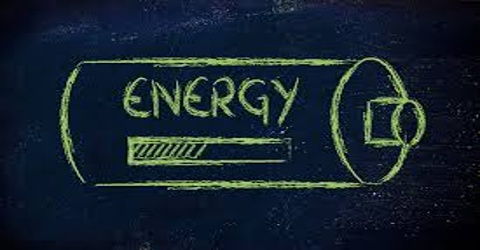# Energy in Thermodynamics

Energy of a system is defined as ‘its capacity to do work’. There are various forms of energy and conversion of energy from one form into another is possible. Electrical energy is converted into mechanical energy with the help of an electric motor. Chemical energy is transformed into mechanical energy in an internal combustion engine, such as that of a motor car or an aeroplane, in a flashlight battery chemical energy of a cell is converted into light energy via electrical energy. Broadly speaking, energy may be classified into two types:

(i) Kinetic Energy and

(ii) Potential Energy.Kinetic Energy: Energy possessed by a system by virtue of its mass and speed is, called kinetic energy. The kinetic energy of a body is measured by the amount of work that the body can do.

Potential Energy: An object possesses potential energy by virtue of its position in a force field (an object above the surface of the earth is under the influence of gravitational force; or when an atom is bonded in a molecule it is under the influence of binding force). An atom bonded with other atoms in a molecule has potential energy.

If the atom is bonded to a different atom, its potential energy will be different. An object above the surface of the earth has potential energy equal to m.g.h (m = mass, h = height above the surface of the earth and g = acceleration due to gravity). Similarly, a coiled spring has potential energy and can do mechanical work, such as dose the minute and second hands in a watch. There are innumerable examples, like this.